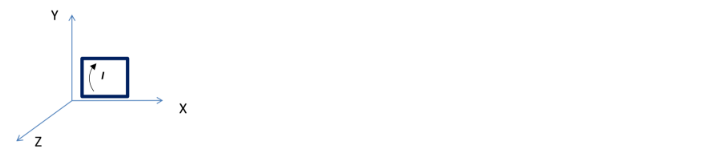# Problem: A copper wire is 8.0 m long and has a cross-sectional area of 1 x 10  -4 m2. The wire forms a loop in the shape of a square and is connected to a battery that applies a potential difference of 10 V such that current flows clockwise (looking down along z-axis) as shown below. The loop is placed on the XY plane in a uniform magnetic field of magnitude 0.5 T directed along the X direction (battery not shown). What is the magnitude of the torque on the loop and its expected direction of rotation? Resistivity of copper is 1.7 x 10-8 Ωm. A. 14706 N.m, rotate about y axis B. 14706 N.m, rotate about z axis C. 24802 N.m rotate about y axis D. 24802 N.m rotate about z axis E. None of the above

###### Problem Details

A copper wire is 8.0 m long and has a cross-sectional area of 1 x 10  -4 m2. The wire forms a loop in the shape of a square and is connected to a battery that applies a potential difference of 10 V such that current flows clockwise (looking down along z-axis) as shown below. The loop is placed on the XY plane in a uniform magnetic field of magnitude 0.5 T directed along the X direction (battery not shown). What is the magnitude of the torque on the loop and its expected direction of rotation? Resistivity of copper is 1.7 x 10-8 Ωm.

A. 14706 N.m, rotate about y axis

B. 14706 N.m, rotate about z axis

C. 24802 N.m rotate about y axis

D. 24802 N.m rotate about z axis

E. None of the above